# How to find top-N records using MapReduce

Finding top 10 or 20 records from a large dataset is the heart of many recommendation systems and it is also an important attribute for data analysis. Here, we will discuss the two methods to find top-N records as follows.

Method 1: First, let’s find out top-10 most viewed movies to understand the methods and then we will generalize it for ‘n’ records.

Data format:

`movie_name and no_of_views (tab separated)`

Approach Used: Using TreeMap. Here, the idea is to use Mappers to find local top 10 records, as there can be many Mappers running parallely on different blocks of data of a file. And then all these local top 10 records will be aggregated at Reducer where we find top 10 global records for the file.

Example: Assume that file(30 TB) is divided into 3 blocks of 10 TB each and each block is processed by a Mapper parallelly so we find top 10 records (local) for that block. Then this data moves to the reducer where we find the actual top 10 records from the file movie.txt.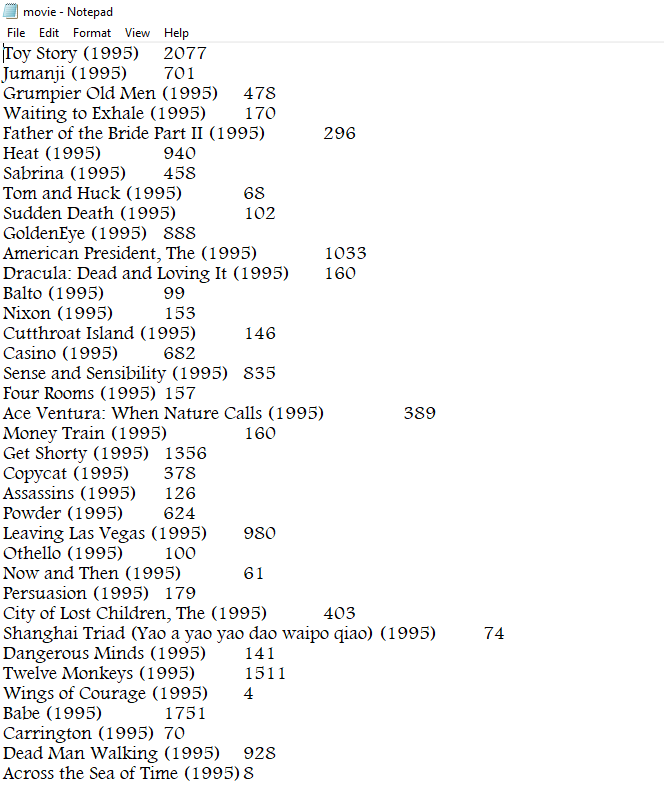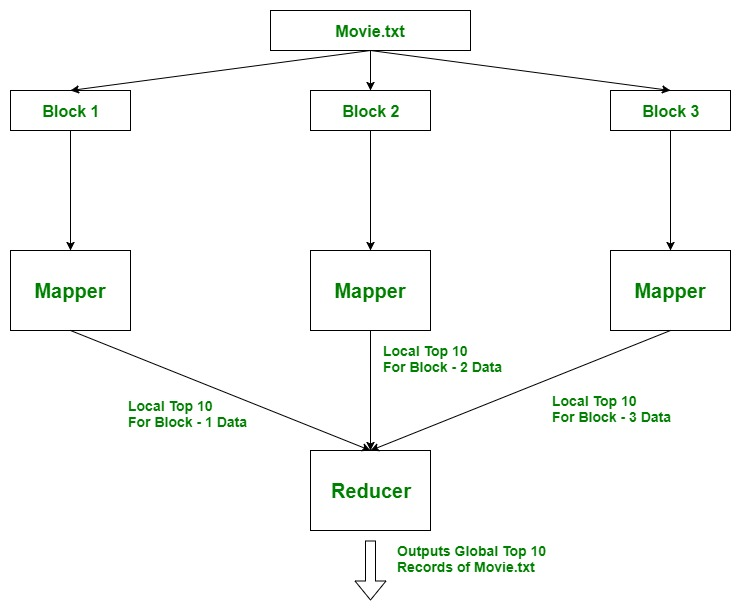Mapper code:

 `import` `java.io.*; ` `import` `java.util.*; ` `import` `org.apache.hadoop.io.Text; ` `import` `org.apache.hadoop.io.LongWritable; ` `import` `org.apache.hadoop.mapreduce.Mapper; ` ` `  `public` `class` `top_10_Movies_Mapper ``extends` `Mapper { ` ` `  `    ``private` `TreeMap tmap; ` ` `  `    ``@Override` `    ``public` `void` `setup(Context context) ``throws` `IOException, ` `                                     ``InterruptedException ` `    ``{ ` `        ``tmap = ``new` `TreeMap(); ` `    ``} ` ` `  `    ``@Override` `    ``public` `void` `map(Object key, Text value, ` `       ``Context context) ``throws` `IOException,  ` `                      ``InterruptedException ` `    ``{ ` ` `  `        ``// input data format => movie_name     ` `        ``// no_of_views  (tab seperated) ` `        ``// we split the input data ` `        ``String[] tokens = value.toString().split(``"\t"``); ` ` `  `        ``String movie_name = tokens[``0``]; ` `        ``long` `no_of_views = Long.parseLong(tokens[``1``]); ` ` `  `        ``// insert data into treeMap, ` `        ``// we want top 10  viewed movies ` `        ``// so we pass no_of_views as key ` `        ``tmap.put(no_of_views, movie_name); ` ` `  `        ``// we remove the first key-value ` `        ``// if it's size increases 10 ` `        ``if` `(tmap.size() > ``10``) ` `        ``{ ` `            ``tmap.remove(tmap.firstKey()); ` `        ``} ` `    ``} ` ` `  `    ``@Override` `    ``public` `void` `cleanup(Context context) ``throws` `IOException, ` `                                       ``InterruptedException ` `    ``{ ` `        ``for` `(Map.Entry entry : tmap.entrySet())  ` `        ``{ ` ` `  `            ``long` `count = entry.getKey(); ` `            ``String name = entry.getValue(); ` ` `  `            ``context.write(``new` `Text(name), ``new` `LongWritable(count)); ` `        ``} ` `    ``} ` `} `

Explanation: The important point to note here is that we use “context.write()” in cleanup() method which runs only once at the end in the lifetime of Mapper. Mapper processes one key-value pair at a time and writes them as intermediate output on local disk. But we have to process whole block (all key-value pairs) to find top10, before writing the output, hence we use context.write() in cleanup().

Reducer code:

 `import` `java.io.IOException; ` `import` `java.util.Map; ` `import` `java.util.TreeMap; ` ` `  `import` `org.apache.hadoop.io.LongWritable; ` `import` `org.apache.hadoop.io.Text; ` `import` `org.apache.hadoop.mapreduce.Reducer; ` ` `  `public` `class` `top_10_Movies_Reducer ``extends` `Reducer { ` ` `  `    ``private` `TreeMap tmap2; ` ` `  `    ``@Override` `    ``public` `void` `setup(Context context) ``throws` `IOException, ` `                                     ``InterruptedException ` `    ``{ ` `        ``tmap2 = ``new` `TreeMap(); ` `    ``} ` ` `  `    ``@Override` `    ``public` `void` `reduce(Text key, Iterable values, ` `      ``Context context) ``throws` `IOException, InterruptedException ` `    ``{ ` ` `  `        ``// input data from mapper ` `        ``// key                values ` `        ``// movie_name         [ count ] ` `        ``String name = key.toString(); ` `        ``long` `count = ``0``; ` ` `  `        ``for` `(LongWritable val : values) ` `        ``{ ` `            ``count = val.get(); ` `        ``} ` ` `  `        ``// insert data into treeMap, ` `        ``// we want top 10 viewed movies ` `        ``// so we pass count as key ` `        ``tmap2.put(count, name); ` ` `  `        ``// we remove the first key-value ` `        ``// if it's size increases 10 ` `        ``if` `(tmap2.size() > ``10``) ` `        ``{ ` `            ``tmap2.remove(tmap2.firstKey()); ` `        ``} ` `    ``} ` ` `  `    ``@Override` `    ``public` `void` `cleanup(Context context) ``throws` `IOException, ` `                                       ``InterruptedException ` `    ``{ ` ` `  `        ``for` `(Map.Entry entry : tmap2.entrySet())  ` `        ``{ ` ` `  `            ``long` `count = entry.getKey(); ` `            ``String name = entry.getValue(); ` `            ``context.write(``new` `LongWritable(count), ``new` `Text(name)); ` `        ``} ` `    ``} ` `} `

Explanation: Same logic as mapper. Reducer processes one key-value pair at a time and writes them as final output on HDFS. But we have to process all key-value pairs to find top10, before writing the output, hence we use cleanup().

Driver Code:

 `import` `org.apache.hadoop.conf.Configuration; ` `import` `org.apache.hadoop.fs.Path; ` `import` `org.apache.hadoop.io.LongWritable; ` `import` `org.apache.hadoop.io.Text; ` `import` `org.apache.hadoop.mapreduce.Job; ` `import` `org.apache.hadoop.mapreduce.lib.input.FileInputFormat; ` `import` `org.apache.hadoop.mapreduce.lib.output.FileOutputFormat; ` `import` `org.apache.hadoop.util.GenericOptionsParser; ` ` `  `public` `class` `Driver { ` ` `  `    ``public` `static` `void` `main(String[] args) ``throws` `Exception ` `    ``{ ` `        ``Configuration conf = ``new` `Configuration(); ` `        ``String[] otherArgs = ``new` `GenericOptionsParser(conf, ` `                                  ``args).getRemainingArgs(); ` ` `  `        ``// if less than two paths  ` `        ``// provided will show error ` `        ``if` `(otherArgs.length < ``2``)  ` `        ``{ ` `            ``System.err.println(``"Error: please provide two paths"``); ` `            ``System.exit(``2``); ` `        ``} ` ` `  `        ``Job job = Job.getInstance(conf, ``"top 10"``); ` `        ``job.setJarByClass(Driver.``class``); ` ` `  `        ``job.setMapperClass(top_10_Movies_Mapper.``class``); ` `        ``job.setReducerClass(top_10_Movies_Reducer.``class``); ` ` `  `        ``job.setMapOutputKeyClass(Text.``class``); ` `        ``job.setMapOutputValueClass(LongWritable.``class``); ` ` `  `        ``job.setOutputKeyClass(LongWritable.``class``); ` `        ``job.setOutputValueClass(Text.``class``); ` ` `  `        ``FileInputFormat.addInputPath(job, ``new` `Path(otherArgs[``0``])); ` `        ``FileOutputFormat.setOutputPath(job, ``new` `Path(otherArgs[``1``])); ` ` `  `        ``System.exit(job.waitForCompletion(``true``) ? ``0` `: ``1``); ` `    ``} ` `} `

Running the jar file:

• We export all the classes as jar files.
• We move our file movie.txt from local file system to /geeksInput in HDFS.
`bin/hdfs dfs -put ../Desktop/movie.txt  /geeksInput`
• We now run the yarn services to run the jar file.
`bin/yarn  jar  jar_file_location  package_Name.Driver_classname   input_path  output_path `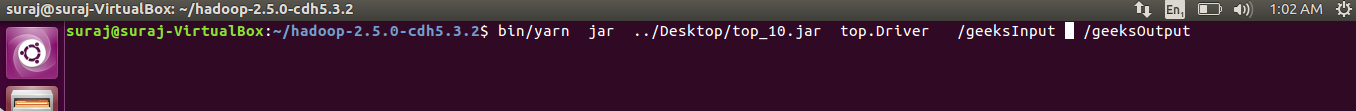• Code running: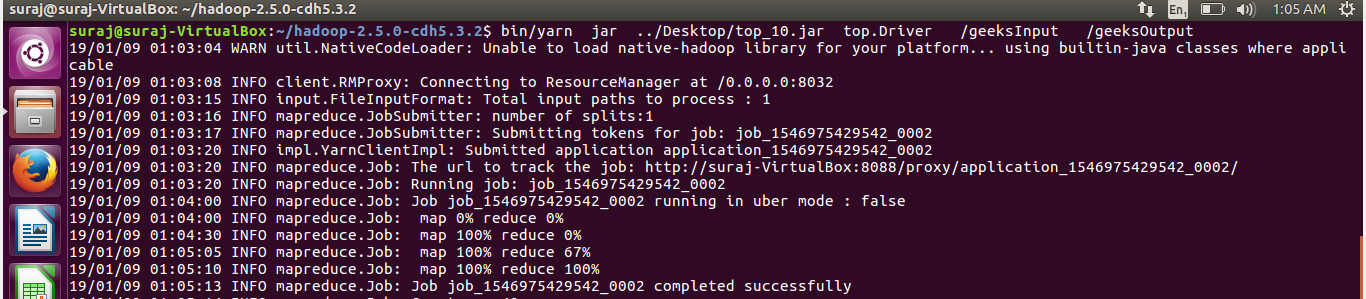Output: In ascending order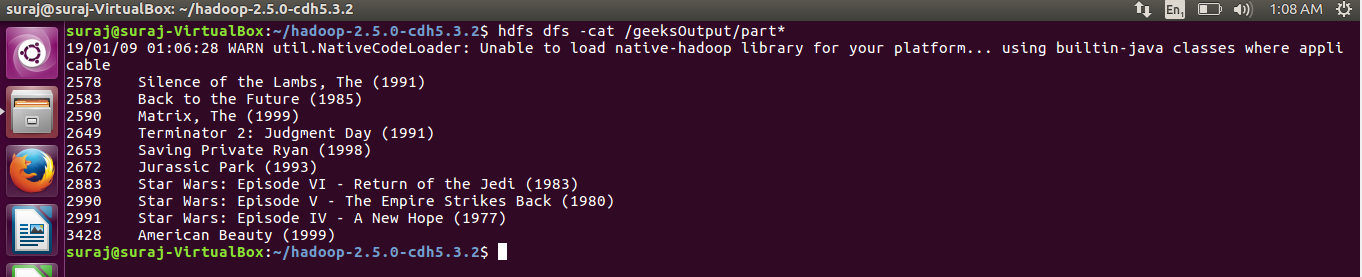Method 2: This method is based on the property that output from Mapper is sorted based on key before going to the reducer. Let’s print in descending order this time. Now to do so we just multiply the key with -1 in mapper, so that after sorting higher numbers appears on top (magnitude wise). And now we just print the 10 records removing the -ve sign from keys.

Example: At reducer

```Keys After sorting:
23
25
28
..
```

If key multiplied with -1

```Keys After sorting:
-28
-25
-23
..
```

Mapper Code:

 `import` `java.io.*; ` `import` `org.apache.hadoop.io.Text; ` `import` `org.apache.hadoop.io.LongWritable; ` `import` `org.apache.hadoop.mapreduce.Mapper; ` ` `  `public` `class` `top_10_Movies2_Mapper ``extends` `Mapper { ` ` `  `    ``// data format  => movie_name      ` `    ``// no_of_views   (tab seperated) ` `    ``@Override` `    ``public` `void` `map(Object key, Text value,  ` `       ``Context context) ``throws` `IOException,  ` `                      ``InterruptedException ` `    ``{ ` ` `  `        ``String[] tokens = value.toString().split(``"\t"``); ` ` `  `        ``String movie_name = tokens[``0``]; ` `        ``long` `no_of_views = Long.parseLong(tokens[``1``]); ` ` `  `        ``no_of_views = (-``1``) * no_of_views; ` ` `  `        ``context.write(``new` `LongWritable(no_of_views), ` `                              ``new` `Text(movie_name)); ` `    ``} ` `} `

Reducer Code:

 `import` `java.io.*; ` `import` `org.apache.hadoop.io.Text; ` `import` `org.apache.hadoop.io.LongWritable; ` `import` `org.apache.hadoop.mapreduce.Reducer; ` ` `  `public` `class` `top_10_Movies2_Reducer ``extends` `Reducer { ` ` `  `    ``static` `int` `count; ` ` `  `    ``@Override` `    ``public` `void` `setup(Context context) ``throws` `IOException, ` `                                     ``InterruptedException ` `    ``{ ` `        ``count = ``0``; ` `    ``} ` ` `  `    ``@Override` `    ``public` `void` `reduce(LongWritable key, Iterable values, ` `      ``Context context) ``throws` `IOException, InterruptedException ` `    ``{ ` ` `  `        ``// key                  values ` `        ``//-ve of no_of_views    [ movie_name ..] ` `        ``long` `no_of_views = (-``1``) * key.get(); ` ` `  `        ``String movie_name = ``null``; ` ` `  `        ``for` `(Text val : values)  ` `        ``{ ` `            ``movie_name = val.toString(); ` `        ``} ` ` `  `        ``// we just write 10 records as output ` `        ``if` `(count < ``10``) ` `        ``{ ` `            ``context.write(``new` `LongWritable(no_of_views), ` `                                  ``new` `Text(movie_name)); ` `            ``count++; ` `        ``} ` `    ``} ` `} `

Explanation: Here, setup() method is the method which runs only once at the beginning
in the life time of a Reducer/Mapper. Since we want to print only 10 records we define the count variable in setup() method.

Driver Code:

 `import` `org.apache.hadoop.conf.Configuration; ` `import` `org.apache.hadoop.fs.Path; ` `import` `org.apache.hadoop.io.LongWritable; ` `import` `org.apache.hadoop.io.Text; ` `import` `org.apache.hadoop.mapreduce.Job; ` `import` `org.apache.hadoop.mapreduce.lib.input.FileInputFormat; ` `import` `org.apache.hadoop.mapreduce.lib.output.FileOutputFormat; ` `import` `org.apache.hadoop.util.GenericOptionsParser; ` ` `  `public` `class` `Driver { ` ` `  `    ``public` `static` `void` `main(String[] args) ``throws` `Exception ` `    ``{ ` `        ``Configuration conf = ``new` `Configuration(); ` `        ``String[] otherArgs = ``new` `GenericOptionsParser(conf ` `                               ``, args).getRemainingArgs(); ` ` `  `        ``// if less than two paths  ` `        ``// provided will show error ` `        ``if` `(otherArgs.length < ``2``)  ` `        ``{ ` `            ``System.err.println(``"Error: please provide two paths"``); ` `            ``System.exit(``2``); ` `        ``} ` ` `  `        ``Job job = Job.getInstance(conf, ``"top_10 program_2"``); ` `        ``job.setJarByClass(Driver.``class``); ` ` `  `        ``job.setMapperClass(top_10_Movies2_Mapper.``class``); ` `        ``job.setReducerClass(top_10_Movies2_Reducer.``class``); ` ` `  `        ``job.setMapOutputKeyClass(LongWritable.``class``); ` `        ``job.setMapOutputValueClass(Text.``class``); ` ` `  `        ``job.setOutputKeyClass(LongWritable.``class``); ` `        ``job.setOutputValueClass(Text.``class``); ` ` `  `        ``FileInputFormat.addInputPath(job, ``new` `Path(otherArgs[``0``])); ` `        ``FileOutputFormat.setOutputPath(job, ``new` `Path(otherArgs[``1``])); ` ` `  `        ``System.exit(job.waitForCompletion(``true``) ? ``0` `: ``1``); ` `    ``} ` `} `

Running the jar file: We now run the yarn services to run the jar file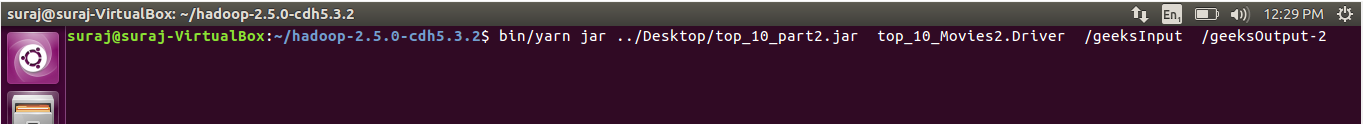Code running: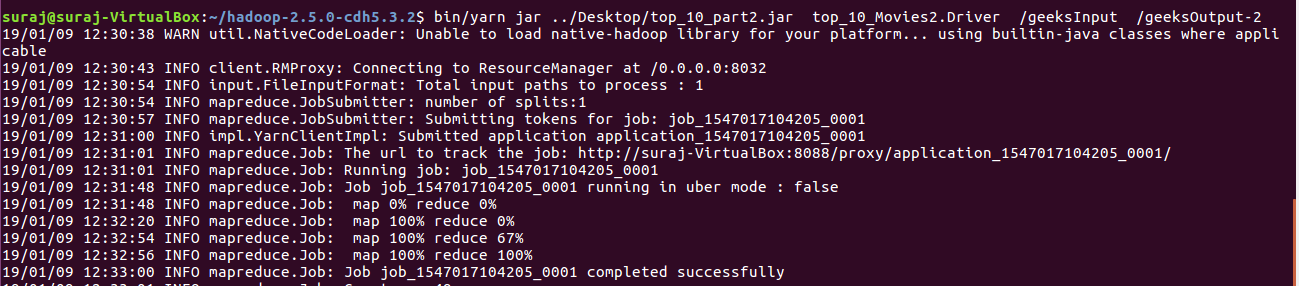Output: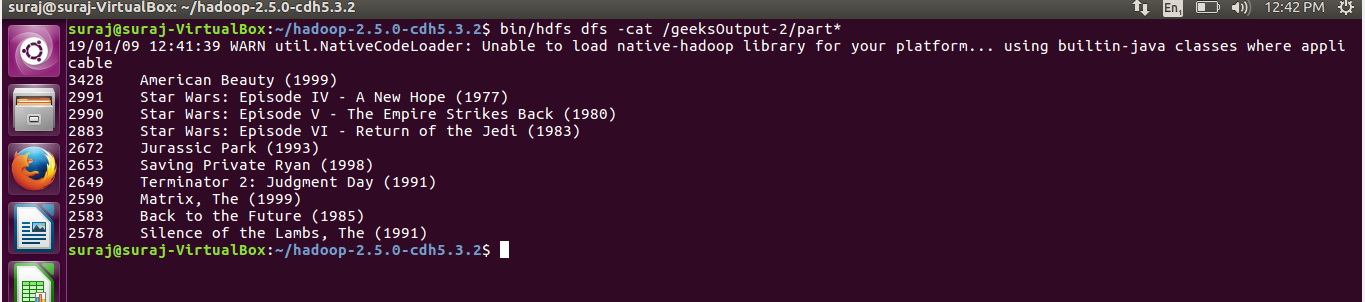Note: One important thing to observe is that though Method-2 is easy to implement but it is not very efficient compared to Method-1 as we are passing all key-value pairs to reducer i.e. there is a lot of data movement which may lead to bottleneck situations. But in Method-1 we are only passing 10 key-value pairs to the reducer.

Generalizing it for ‘n’ records: Lets modify our second program for some ‘n’ records whose value we may pass at runtime. First some points to observe:

• We make our custom parameter using set() method
`configuration_object.set(String name, String value)`
• This value can be accessed in any Mapper/Reducer by using get() method
```Configuration conf = context.getConfiguration();

// we will store value in String variable
String  value = conf.get(String name);
```
• Mapper code: The Mapper code will remain same as we are not using the value there.

Reducer code: Here we make some changes in the setup() method.

 `import` `java.io.IOException; ` `import` `org.apache.hadoop.io.LongWritable; ` `import` `org.apache.hadoop.io.Text; ` `import` `org.apache.hadoop.mapreduce.Reducer; ` `import` `org.apache.hadoop.conf.Configuration; ` ` `  `public` `class` `top_n_Reducer ``extends` `Reducer { ` ` `  `    ``static` `int` `count; ` ` `  `    ``@Override` `    ``public` `void` `setup(Context context) ``throws` `IOException, ` `                                     ``InterruptedException ` `    ``{ ` ` `  `        ``Configuration conf = context.getConfiguration(); ` ` `  `        ``// we will use the value passed in myValue at runtime ` `        ``String param = conf.get(``"myValue"``); ` ` `  `        ``// converting the String value to integer ` `        ``count = Integer.parseInt(param); ` `    ``} ` ` `  `    ``@Override` `    ``public` `void` `reduce(LongWritable key, Iterable values, ` `     ``Context context) ``throws` `IOException, InterruptedException ` `    ``{ ` ` `  `        ``long` `no_of_views = (-``1``) * key.get(); ` `        ``String movie_name = ``null``; ` ` `  `        ``for` `(Text val : values) { ` `            ``movie_name = val.toString(); ` `        ``} ` ` `  `        ``// we just write 10 records as output ` `        ``if` `(count > ``0``) ` `        ``{ ` `            ``context.write(``new` `LongWritable(no_of_views), ` `                                  ``new` `Text(movie_name)); ` `            ``count--; ` `        ``} ` `    ``} `

Driver Code:

 `import` `org.apache.hadoop.conf.Configuration; ` `import` `org.apache.hadoop.fs.Path; ` `import` `org.apache.hadoop.io.LongWritable; ` `import` `org.apache.hadoop.io.Text; ` `import` `org.apache.hadoop.mapreduce.Job; ` `import` `org.apache.hadoop.mapreduce.lib.input.FileInputFormat; ` `import` `org.apache.hadoop.mapreduce.lib.output.FileOutputFormat; ` `import` `org.apache.hadoop.util.GenericOptionsParser; ` ` `  `public` `class` `genericDriver { ` ` `  `    ``public` `static` `void` `main(String[] args) ``throws` `Exception ` `    ``{ ` `        ``Configuration conf = ``new` `Configuration(); ` ` `  `        ``/* here we set our own custom parameter  myValue with  ` `         ``* default value 10. We will overwrite this value in CLI ` `         ``* at runtime. ` `         ``* Remember that both parameters are Strings and we  ` `         ``* have convert them to numeric values when required. ` `         ``*/` ` `  `        ``conf.set(``"myValue"``, ``"10"``); ` ` `  `        ``String[] otherArgs = ``new` `GenericOptionsParser(conf, ` `                                  ``args).getRemainingArgs(); ` ` `  `        ``// if less than two paths provided will show error ` `        ``if` `(otherArgs.length < ``2``)  ` `        ``{ ` `            ``System.err.println(``"Error: please provide two paths"``); ` `            ``System.exit(``2``); ` `        ``} ` ` `  `        ``Job job = Job.getInstance(conf, ``"top_10 program_2"``); ` `        ``job.setJarByClass(genericDriver.``class``); ` ` `  `        ``job.setMapperClass(top_n_Mapper.``class``); ` `        ``job.setReducerClass(top_n_Reducer.``class``); ` ` `  `        ``job.setMapOutputKeyClass(LongWritable.``class``); ` `        ``job.setMapOutputValueClass(Text.``class``); ` ` `  `        ``job.setOutputKeyClass(LongWritable.``class``); ` `        ``job.setOutputValueClass(Text.``class``); ` ` `  `        ``FileInputFormat.addInputPath(job, ``new` `Path(otherArgs[``0``])); ` `        ``FileOutputFormat.setOutputPath(job, ``new` `Path(otherArgs[``1``])); ` ` `  `        ``System.exit(job.waitForCompletion(``true``) ? ``0` `: ``1``); ` `    ``} `

Now comes the most important part i.e. passing a value to our custom parameter myValue from CLI. We use -D command line option => as shown below:

```-D  property=value  (Use value for given property.)
```

Lets pass 5 as value to find top 5 records: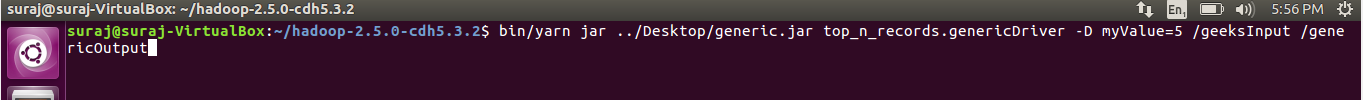Output: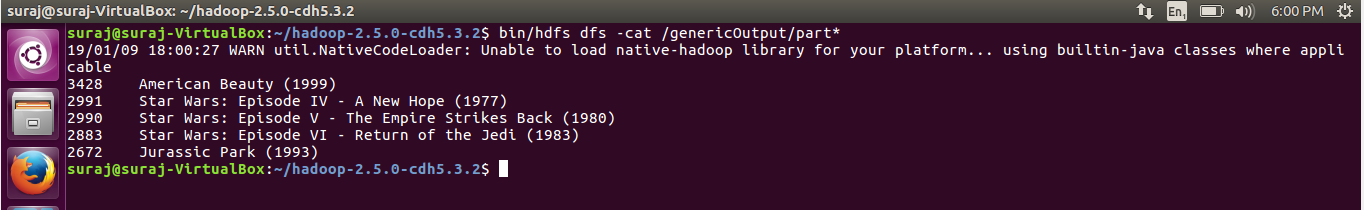My Personal Notes arrow_drop_upA polyglot and a Big Data enthusiast

If you like GeeksforGeeks and would like to contribute, you can also write an article using contribute.geeksforgeeks.org or mail your article to contribute@geeksforgeeks.org. See your article appearing on the GeeksforGeeks main page and help other Geeks.

Please Improve this article if you find anything incorrect by clicking on the "Improve Article" button below.

Article Tags :
Practice Tags :

1

Please write to us at contribute@geeksforgeeks.org to report any issue with the above content.